# metal

Conductor material

Since R2021a

## Syntax

``m = metal(material)``
``m = metal(Name,Value)``

## Description

example

````m = metal(material)` creates a metal with predefined characteristics and stores it as a metal object. Use this metal as the conductor material for the antenna or array catalog elements. You can specify a material from the `MetalCatalog`. The default value for material is perfect electric conductor (PEC).```

example

````m = metal(Name,Value)` creates the metal object based on the properties specified by one or more Name-Value Arguments.```

## Examples

collapse all

Use steel as the as a conductor for a monocone antenna.

`m = metal("Steel")`
```m = metal with properties: Name: 'Steel' Conductivity: 6990000 Thickness: 6.8000e-04 For more materials see catalog ```

Create a monocone antenna using the `monocone` antenna object.

`ant = monocone(Conductor=m)`
```ant = monocone with properties: Radii: [5.0000e-04 0.0110 0.0110] GroundPlaneRadius: 0.0325 ConeHeight: 0.0115 Height: 0.0250 FeedHeight: 5.0000e-04 FeedWidth: 5.0000e-04 Conductor: [1x1 metal] Tilt: 0 TiltAxis: [1 0 0] Load: [1x1 lumpedElement] ```

View the antenna using the `show`` `function.

`show(ant)`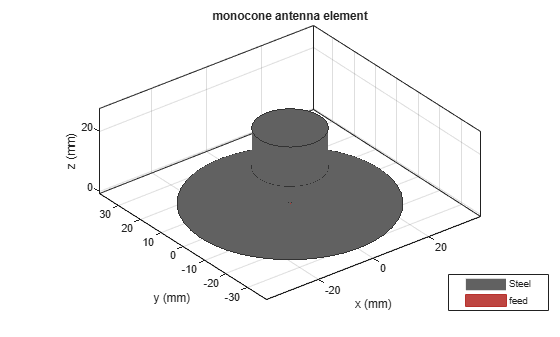Create an annealed copper conductor with the conductivity of 5.8001e07 S/m and thickness of 1e-04 m.

`m = metal(Name="Annealed Copper",Conductivity=5.8001e07,Thickness=1e-04)`
```m = metal with properties: Name: 'Annealed Copper' Conductivity: 58001000 Thickness: 1.0000e-04 For more materials see catalog ```

Create a birdcage antenna using the annealed copper conductor.

`ant = birdcage(Conductor=m)`
```ant = birdcage with properties: NumRungs: 16 CoilRadius: 0.4000 CoilHeight: 0.0400 RungHeight: 0.4600 ShieldRadius: 0 ShieldHeight: 0 Phantom: [] FeedLocations: [2x3 double] FeedVoltage: 1 FeedPhase: 0 Conductor: [1x1 metal] Tilt: 0 TiltAxis: [1 0 0] Load: [1x1 lumpedElement] ```

View the antenna using `show`` `function.

`show(ant)`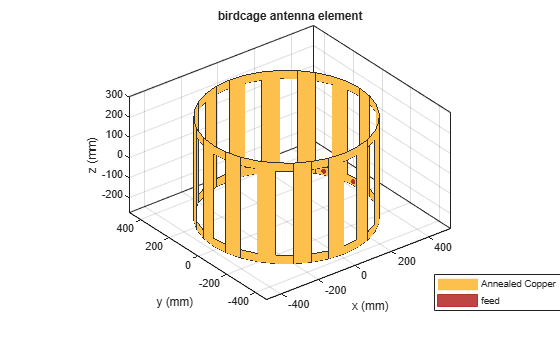Create and visualize the microstrip patch antennas with PEC, copper, silver, and aluminium metals as conductors.

```ant_patchPEC = patchMicrostrip(Substrate=dielectric("FR4"),Conductor=metal("PEC")); show(ant_patchPEC)```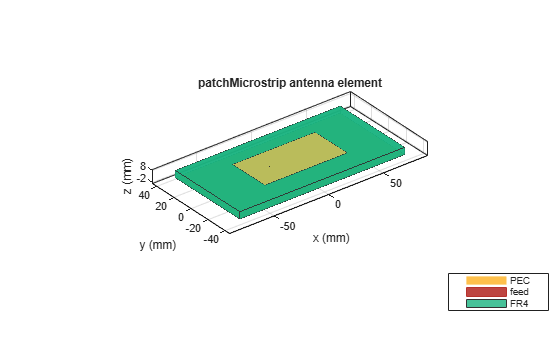```ant_patchCopper = patchMicrostrip(Substrate=dielectric("FR4"),Conductor=metal("Copper")); show(ant_patchCopper)```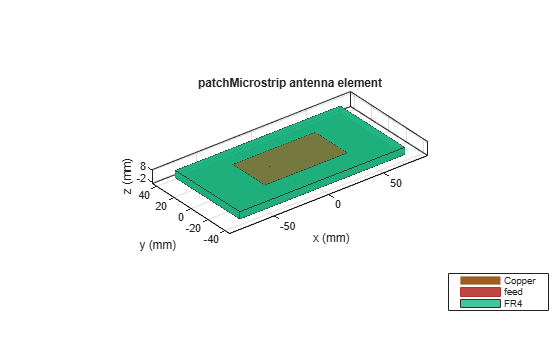```ant_patchSilver = patchMicrostrip(Substrate=dielectric("FR4"),Conductor=metal("Silver")); show(ant_patchSilver)```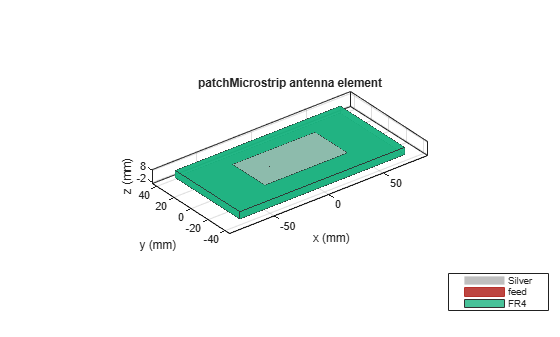```ant_patchAluminium = patchMicrostrip(Substrate=dielectric("FR4"),Conductor=metal("Aluminium")); show(ant_patchAluminium)```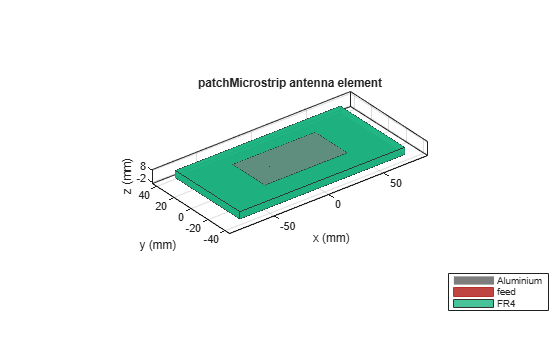Compare the impedance values at a frequency of 1.2 GHz.

`Z_patchPEC = impedance(ant_patchPEC,1.2e09)`
```Z_patchPEC = 1.2507 +17.6064i ```
`Z_patchCopper = impedance(ant_patchCopper,1.2e09)`
```Z_patchCopper = 1.2904 +17.6489i ```
`Z_patchSilver = impedance(ant_patchSilver,1.2e09)`
```Z_patchSilver = 1.6159 +17.6220i ```
`Z_patchAluminium = impedance(ant_patchAluminium,1.2e09)`
```Z_patchAluminium = 1.3006 +17.6598i ```

## Input Arguments

collapse all

Metal material, specified as a metal object from the `MetalCatalog`. The default material is PEC having infinite conductivity and zero thickness.

Example: `"Iron"`

Data Types: `string`

### Name-Value Arguments

Specify optional pairs of arguments as `Name1=Value1,...,NameN=ValueN`, where `Name` is the argument name and `Value` is the corresponding value. Name-value arguments must appear after other arguments, but the order of the pairs does not matter.

Example: `Name="Iron"`

Before R2021a, use commas to separate each name and value, and enclose `Name` in quotes.

Example: `'Name','Iron'`

Name of the metal material to use as a conductor, specified as a string.

Example: `"Tungsten"`

Data Types: `string`

Conductivity of the metal material, specified as a scalar in Siemens per meters(S/m). If you set `Conductivity` to `Inf`, you must set `Thickness` to 0. The minimum value of conductivity is 1e05 S/m in the Antenna Toolbox™

Example: `4.8e06`

Data Types: `double`

Thickness of the metal material along the default z-axis, specified as a scalar in meters. The upper limit of thickness value is 1e-03 m in the Antenna Toolbox

Example: `0.26e-6`

Data Types: `double`

## Output Arguments

collapse all

Conductor metal with predefined properties, returned as a metal object. You can use the `metal` object as a conductor for the antenna catalog elements.

## Version History

Introduced in R2021a